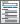Home  /  Products  /  Features  /  Choice models

## Choice models

Model your discrete-choice data—say, a choice to travel by bus, train, car, or airplane—with a conditional logit, multinomial probit, or mixed logit model. Is your outcome instead a ranking of preferred travel methods? Fit a rank-ordered probit or rank-ordered logit model. Regardless of the model fit, you can use margins to easily interpret the results. Estimate how much wait times at the airport affect the probability of traveling by air or even by train.

Discrete-choice data

• Alternative-specific and case-specific covariates
• Balanced and unbalanced choice sets
• One selected outcome per case or ranked outcomes

Conditional logit models• Odds ratios and relative-risk ratios
• Robust, cluster–robust, bootstrap, and jackknife standard errors

Multinomial probit models• Homo- or heteroskedastic variances
• Various correlation structures, including user-specified
• Relaxes IIA assumption
• Probabilities based on GHK simulator
• Robust, cluster–robust, bootstrap, and jackknife standard errors

Nested logit modelsSummarize choice data• Tabulate choice sets
• Summarize covariates by alternative
• Tabulate covariates by chosen alternative
• Report potential problems in data

Rank-ordered probit models• Plackett–Luce model, exploded logit, choice-based conjoint analysis
• Homo- or heteroskedastic variances
• Various correlation structures, including user-specified
• Relaxes IIA assumption
• Probabilities based on GHK simulator
• Robust, cluster–robust, bootstrap, and jackknife standard errors

Rank-ordered logit models• Also known as
• Plackett–Luce model
• Exploded logit
• Choice-based conjoint analysis
• Complete rankings of ordered outcome
• Incomplete rankings of ordered outcome
• Account for ties (indifference)
• Prediction of probability that alternatives are ranked first
• Robust, cluster–robust, bootstrap, and jackknife standard errors

Truly interpret results• Estimate
• Expected probabilities of selecting each alternative
• In the population
• In a subpopulation
• At specified covariate levels
• Difference in probabilities of selecting an alternative
• As a covariate changes for this alternative
• As a covariate changes for another alternative
• As a covariate changes for all alternatives
• Marginal effects
• Tests and confidence intervals for everything